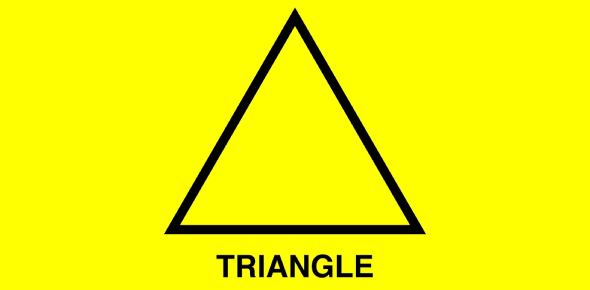# Triangles Vocabulary Quiz

9 Questions | Total Attempts: 131SettingsYou know that a triangle is a shape with three sides and three angles, hence the name, but what other vocabulary are you aware of with regards to the geometrical image? Let’s see how much you really know about triangles.

• 1.
All acute angles
• A.

Acute Triangle

• B.

Equalateral Triangle

• C.

Scalene Triangle

• D.

Obtuse Triangle

• 2.
Has one right angle
• A.

Measure of Three Angles

• B.

Equalateral Triangle

• C.

Right Triangle

• D.

Polygons

• 3.
Has one obtuse angle
• A.

Scalene Triangle

• B.

Obtuse Triangle

• C.

Isosceles Triangle

• D.

Equalateral Triangle

• 4.
A triangle in which at least 2 sides are congruent
• A.

Scalene Triangle

• B.

Equalateral Triangle

• C.

Isosceles Triangle

• D.

Polygons

• 5.
A triangle having all three sides congruent and all three angles congruent
• A.

Equalateral Triangle

• B.

Measure of Three Angles

• C.

Scalene Triangle

• D.

Polygons

• 6.
A triangle in which no sides are congruent
• A.

Polygons

• B.

Equalateral Triangle

• C.

Isosceles Triangle

• D.

Scalene Triangle

• 7.
A simple closed figure that does not curve or intersect formed by three or more line segments
• A.

Right Triangle

• B.

Measure of Three Angles

• C.

Polygons

• D.

Triangle

• 8.
A polygon with three sides
• A.

Obtuse Triangle

• B.

Right Triangle

• C.

Acute Triangles

• D.

Triangle

• 9.
180 degrees
• A.

Triangle

• B.

Isosceles Triangle

• C.

Measure of Three Angles

• D.

Polygons

Related Topics